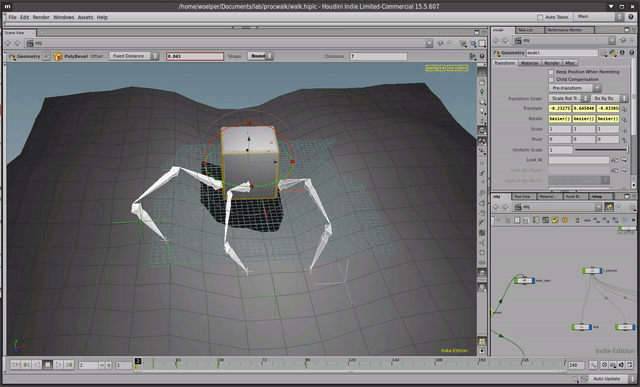I wanted to do something like this really badly in Maya once. Tried in Houdini for fun, and turns out something like this is easy to do and very entertaining!The first interation was super simple and basically the idea that the position of each leg snapped to some rounded position of the leg.

The awesome detail about this is that there is no playback happening. This all happens on a static timeline in real time.

The logic now takes ground geo into account and uses frame 1 as a 'pose frame' similar to the capture frame approach. That means that you could keyframe the 'normal' leg positions relative to the body in frame 1, keyframe them and start moving the whole stuff in the other frames.

TODO:
Expose more parameters
If one would animate the base geo, stuff like wiggle or lag could greatly add to the overall animation. As of now, on every frame != 1 the legs will be keyframed, making CHOP effects possible.

``````import hou
import math

# /////////////////////////////////////// utils
def lerp(t, a, b):
return a + t * (b - a)

def roundVec(v, fac, seed):
#seed = random.random()*0.1
seed = 0.1 * seed - 0.05 * seed
x = (round(v * fac + seed)) / fac
y = (round(v * fac + seed)) / fac
z = (round(v * fac + seed)) / fac
return hou.Vector3(x, y, z)

def find_nearest(v, node, maxdist=3):
# TODO probably take the nearest topmost point
geo = node.displayNode().geometry()
nearest = [maxdist, v]
for p in geo.points():
dist = p.position().distanceTo(v)
if dist < nearest:
nearest = dist
nearest = p.position()
return nearest

# /////////////////////////////////////// setup
def leg_capturepos(leg):
p = leg.capture_pos
t = hou.hmath.buildTranslate(p, p, p)
return t * PARENT_WT

def place_legs():
for no, leg in enumerate(LEGS):
# print leg.name(), no
p = leg_capturepos(leg)
leg.restpos = p.extractTranslates()

def move_legs_gnd():
# distribute_legs()
place_legs()

for no, leg in enumerate(LEGS):
lt = hou.Vector3(leg.parmTuple("t").eval())
next_p = find_nearest(lt, GROUND)
dist = next_p.distanceTo(leg.restpos)
if dist > COMFORT_LENGTH:
# print 'setting', leg
next_restp = find_nearest(leg.restpos, GROUND)
t = hou.hmath.buildTranslate(
next_restp, next_restp, next_restp)
leg.setParmTransform(t)
else:
pass
#t = hou.hmath.buildTranslate(next_p, next_p, next_p)
# leg.setParmTransform(t)

def remove_keyframes():
if hou.frame() == 1:
"""
delete all keyframes if rewound
(@ frame 1)
"""
for leg in LEGS:
parm = leg.parmTuple("t")
parm.deleteAllKeyframes()

def set_keys():
"""
Set keyframe for all legs
"""
frame = hou.frame()

if frame == CAPTURE_FRAME:
return

for leg in LEGS:
pos = hou.Vector3(leg.parmTuple("t").eval())
kfx = hou.Keyframe()
kfx.setFrame(frame)
kfx.setValue(pos)
kfy = hou.Keyframe()
kfy.setFrame(frame)
kfy.setValue(pos)
kfz = hou.Keyframe()
kfz.setFrame(frame)
kfz.setValue(pos)
tchanx = hou.parm('../' + leg.name() + '/tx')
tchany = hou.parm('../' + leg.name() + '/ty')
tchanz = hou.parm('../' + leg.name() + '/tz')
tchanx.setKeyframe(kfx)
tchany.setKeyframe(kfy)
tchanz.setKeyframe(kfz)

# /////////////////////////////////////// globals
THIS_NODE = hou.pwd()
PARENT = THIS_NODE.inputs()
LEGS = hou.node('../l_parents').outputs()
GROUND = hou.node('../ground')
CAPTURE_FRAME = 1
PARENT_WT = PARENT.worldTransform()
COMFORT_LENGTH = 1

def main():
# This is our 'capture' frame

if hou.frame() == CAPTURE_FRAME:
"""
Basically, do nothing in the capture frame.
Just store the actual leg position in the up vector
to access it in any other frame as the capture position.
"""
for leg in LEGS:
# we use the up vector to store the capture pose per leg
pos = hou.Vector3(leg.parmTuple("t").eval())
leg.parmTuple('up').set(pos)
print leg, pos
leg.capture_pos = pos
# move_legs_gnd()
else:
# do the dance
for leg in LEGS:
p = hou.Vector3(leg.parmTuple("up").eval())
leg.capture_pos = p
move_legs_gnd()
set_keys()

main()
``````